Introduction To Mass Transfer Operation

# Introduction To Mass Transfer Operation Notes | Study Mass Transfer - Chemical Engineering

## Document Description: Introduction To Mass Transfer Operation for Chemical Engineering 2022 is part of Mass Transfer preparation. The notes and questions for Introduction To Mass Transfer Operation have been prepared according to the Chemical Engineering exam syllabus. Information about Introduction To Mass Transfer Operation covers topics like and Introduction To Mass Transfer Operation Example, for Chemical Engineering 2022 Exam. Find important definitions, questions, notes, meanings, examples, exercises and tests below for Introduction To Mass Transfer Operation.

Introduction of Introduction To Mass Transfer Operation in English is available as part of our Mass Transfer for Chemical Engineering & Introduction To Mass Transfer Operation in Hindi for Mass Transfer course. Download more important topics related with notes, lectures and mock test series for Chemical Engineering Exam by signing up for free. Chemical Engineering: Introduction To Mass Transfer Operation Notes | Study Mass Transfer - Chemical Engineering
 1 Crore+ students have signed up on EduRev. Have you?

1.1 INTRODUCTION TO MASS TRANSFER OPERATION

Mass transfer is a transport of components under a chemical potential gradient. The component moves to the direction of reducing concentration gradient. The transport occurs from a region of higher concentration to lower concentration. Equilibrium is reached when the gradient is zero. The transport or migration of one constituent from a region of higher concentration to that of a lower concentration is known as mass transfer.
Mass transfer operations depend on molecules diffusing from one distinct phase to another and are based upon differences in the physico-chemical properties of the molecules, such as vapour pressure or solubility. For interphase mass transfer, there is a concentration gradient between bulk and interface, however under steady state, at interface equilibrium is assumed.
Mass transfer operation plays an important role in many industrial processes. A group of operations for separating the components of mixtures is based on the transfer of material from one homogeneous phase to another. These methods is covered by the term mass transfer operations which include techniques like gas absorption and stripping, liquid-liquid extraction, leaching, distillation, humidification, drying, crystallization and number of other separation techniques.

Absorption and stripping- Absorption refers to an operation in which the transfer of material is from a gas phase to a liquid phase. A gas is absorbed by means of liquid in which the solute gas is more or less soluble from its mixture with an inert gas as well as more or less insoluble gas. The liquid is essentially immiscible in the gas phase. The stripping is the separation of gas solute from the liquid phase. The separation of ammonia from an air-ammonia mixture by means of water is a typical example of absorption. The solute is then recovered from the solution by distillation. Depending upon situation, both the fluids (absorbent and absorbing fluid) are reprocessed and/or reused for the operation.

Adsorption and desorption –It exploits the ability of transfer of mass from either a gas or a liquid to the surface of a solid. The adsorption is not a true inter-phase mass transfer operation because the fluid adheres to the solid surface instead of dissolving in the solid. A desorption involves the transfer of mass from the solid surface (adsorbents) to the gas or liquid medium (adsorbates). A few operations of practical applications are (a) elimination of toxic gases and deodorization of air, (b) recovery of solvents, (c) removal of ions from solution, as in demineralization of water, (d) fractionation by selective adsorption of gases, vapours from gases, vapors from vapors and liquids from liquids and many other applications.

Extraction- It refers to a separation of the constituents of a liquid solution by contact with another insoluble liquid. The liquid which is added to the solution to bring about the extraction is known as the solvent. The solution which is to be extracted is called the feed. The solvent-richen product of the operation is called the extract and the residual liquid from which the solute is separated is called the raffinate. The separation of aromatics from kerosene based fuel oils, the production of fuels in the nuclear industry and the separation of penicillin from fermentation mixtures are examples of techniques of extraction.

Leaching- is a treatment of a finely divided solid with a liquid. Some examples of leaching operations are oilseed extraction, extraction of sugar beets with hot water and extraction of medicinal compounds from plant roots, leaves and stems.

Distillation-It is an operation whereby a liquid mixture of miscible and volatile substances is separated into individual components or into group of components by partial vaporization. The separation of a mixture of methanol and water into its components, and separation of petroleum crude into gasoline, kerosene, fuel oil and lubricating stock are examples of distillation process. Humidification and dehumidification- In humidification, the enrichment of vapour content in a gas stream takes place by passing the gas over a liquid. The transfer of water from the liquid phase to the gas phase of a mixture of air and water vapour is a widespread application of humidification. Dehumidification involves the transfer of water vapour from the gas phase to the liquid phase. Removal of water vapour from air by condensation of a cold surface and condensation of carban tetrachloride out of a stream of nitrogen are examples of dehumidification.

Drying and Evaporation- Drying refers to removal of relatively small amount of water or other liquid from a solid material whereas evaporation refers to the removal of relatively large amount of water from solutions. In evaporation the water is removed as vapour at its boiling point. Drying involves the removal of water at temperature below the boiling point by circulating air or some other carrier gas over the material.

Crystallization- Crystallization is a process of formation of solid from a liquid solution based on difference in solute concentration and its solubility at a certain temperature. In crystallization, transfer of a solute occurs from the liquid solution to a pure solid crystalline phase. When solute concentration becomes higher than its solubility at certain temperature, the solute comes out of the solution and forms a crystal.

Membrane separation- This process predominately involves the diffusion of a solute from a liquid or gas through a semi- permeable or microporous membrane to another fluid. Separation of components of the original solution takes place by selectively controlling their passage from one side of membrane to the other. An example of membrane mediated, liquid-liquid separation process is called dialysis. In this process, the microporous membrane allows solutes of low molecular weight in one liquid phase to diffuse readily through it to a second liquid phase by virtue of concentration differences.
Passage of larger colloidal particles through the membrane is difficult. For example, aqueous beet-sugar solutions containing undesired colloidal material are removed by contact with water through a semi-permeable membrane. Sugar and water diffuse through the membrane but not the colloid. If a membrane is placed between a solution and a pure solvent, the solvent diffuses into the solution by osmosis. A reverse pressure, greater than osmotic pressure, is imposed, causing the solvent to flow in the reverse direction, as in the desalination of sea water or brackish water to produce fresh water is called reverse osmosis.

1.2 Concentrations and Flux
1.2.1 Concentrations The concentration of particular species is expressed in variety of ways. In mass transfer operation, the concentration gradient is the driving force when other driving forces (temperature, pressure gradients, etc.) are kept constant. The actual driving force for mass transfer to occur is to create gradient of chemical potential (between two points) which is a function of all external forces. The concentration gradients are generally expressed in terms of mass concentration of component, molar concentration of component and mass or mole fraction of species.

Mass concentration
The mass concentration of species i is expressed as  pi . It is defined as the mass of i per unit volume of a multi-component mixture; that is: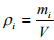(1.1)
It has the same unit as density. Total mass concentration within a mixture is equal to overall density which can be expressed as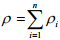(1.2)
where n is the number of species in the mixture.

Mass fraction
The mass fraction of species i is defined by the ratio of mass concentration of species i to the total mass density. It can be expressed as: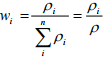(1.3)
From Equations (1.2) and (1.3), it is shown that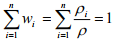(1.4)
Molar Concentration
The molar concentration of component is denoted by Ci. It is defined as moles of ith component per unit volume of mixture. The total concentration in the system can be obtained by sum up all molar concentrations for all species which can be represented as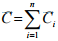(1.5)
Sometimes it is required to convert from mass to molar concentration. This can be accomplished by dividing the mass concentration of species i by the molar weight of that species. For an ideal gas mixture the molar concentration of species i can be obtained from the ideal gas law (PV = nRT) as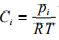(1.6)
where pi is the partial pressure of species i in the mixture, T is the absolute temperature and R is the universal gas constant. So total concentration in the gaseous system can be represented by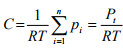(1.7)
where Pt is the total pressure of the system which is sum of partial pressures of all components.
Mole fraction The mole fraction of species i is found by dividing the molar concentration of species i by total concentration in the system which is expressed as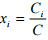for liquid and solid                         (1.8)
and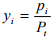for gases                                     (1.9)

If it is summed over all species, then one can get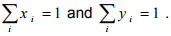Example Problem 1.1: Calculate the mass concentration of 2 gram moles of sulpher dioxide to be used to manufacture sulfuric acid in a 0.20 m3 of reactor.
Solution 1.1: 2 moles of sulpher dioxide is equal to 642g = 128 g So mass concentration is 128 g/0.20 m3 = 1280.001 kg/0.20 m3 = 0.64 kg/m3

Example Problem 1.2: A mixture of noble gases [helium, argon, krypton, and xenon] is at a total pressure of 100 kPa and a temperature of 200 K. If the mixture has equal kmole fractions of each of the gases, determine:
a) The composition of the mixture in terms of mass fractions.
b) Total molar concentration
c) The mass density.
Solution 1.2:
(a) Let 100 kmol of the mixture Molecular weight of helium, argon, krypton (3), and xenon (4) are 4, 40, 83.8 and 131.3 kg/mol respectively.
Moles of each component of the mixture = 25 moles
So total mass of mixture = 254+ 2540+2583.8+25131.3 = 6477.5 kg
So mass fractions (component mass/ Total mass) are:
Helium = 254/6477.5 = 0.015,
Argon = 2540/6477.5= 0.154,
Krypton = 2583.8/6477.5 = 0.323 and
Xenon =25131.3/6477.5 = 0.507 The average molecular weight of the mixture = total mass/100 kmol = 6477.5 kg/100 kmol = 64.775 kg/kmol = 0.064775 kg/mol
(b) Temperature T = 200 K, Pressure P = 100 kPa, R = 8.314 kPa.m3 .kmol-1 .K-1 .
So Total molar concentration, C = P/RT = 60.14 mol/m3 .
(c) Mass density = Total molar concentration (C)  average molecular weight of the mixture = 60.14 mol/m3 0.064775 kg/mol = 3.896 kg/m3

1.2.2 Fluxes
The flux is defined as the rate of transport of species i per unit area in a direction normal to the transport. The flux is calculated with respect to a fixed reference frame. The molar flux of species i can be represented as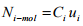(1.10)
Here Ni-molar is the molar flux of species i and ui is the velocity of i with respect to a fixed reference frame. In the same fashion, a mass flux, Ni-mass can be represented as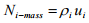(1.11)
The units of the molar and mass fluxes are moles/m2 .s and mass/m2 .s. Sometimes it is convenient to interpret the total flux of species i with respect to an arbitrary reference frame rather than a fixed set of reference frame. The molar flux of species i based on arbitrary reference velocity u0 is denoted by Ji-mol which can be defined as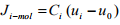(1.12)
Similarly mass flux of species i based on arbitrary reference velocity u0 is denoted by Ji-mass which can be expressed as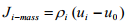(1.13)
In a system, since several molecular species move with different average velocities, a frame of moving reference must be chosen. The important moving references are mass average, molar average and volume average velocities.

Mass-average velocity
The mass average velocity can be defined in terms of the mass concentration and the velocity of species i based on fixed axis. It is expressed as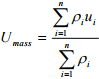(1.14)

Molar average velocity
The molar average velocity can be expressed by the expression analogous to the mass average velocity. It can be represented by replacing mass concentration of species i, pi with molar concentration of species i, Ci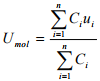(1.15)

Volume average velocity
For experimental analysis the volume average velocity is important due to a fixed system of constant volume. The volume average velocity can be expressed by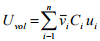(1.16)
where  vi is the partial molar volume of species i.
Relation between fluxes
One can obtained the molar flux of i expressed in Equation (1.12) with respect to the molar average velocity as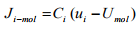(1.17)
Substituting the Equation (1.10) into the Equation (1.17) and rearranging gibes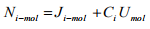(1.18)
Again substituting the definition of molar average velocity defined in Equation (1.15) into the Equation (1.18) we get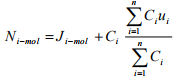(1.19)
Or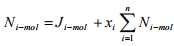(1.20)
For binary system of component A and B,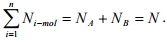Therefore the Equation (1.20) can be written for binary system as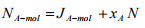(1.21)
Example Problem 1.3: One kmole of gas mixture at a total pressure of 250 kPa and 303 K contains 10% CH4, 30% C2H6, and 60% H2 by volume. The absolute velocities of each species are -10 m/s, -5 m/s, and 15 m/s, respectively, all in the direction of the z-axis.
(a) Determine the molar average velocity, Umol for the mixture.
(b) Evaluate the four fluxes: JCH4-mol, NCH4-mol.

Solution 1.3:
(a)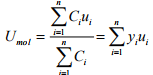=(0.1)(-10)+(0.30)(-5) (0.60)(15)=6.5 m/s
(b) C = P/RT = 250/(8.314*303) = 0.09924 kmol/m3 = 99.24 mol/m3
CCH4 = 0.10*99.24 mol/m3 = 9.924 mol/m3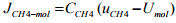= (9.924)*(-10-6.5) = -163.746 mol/m2s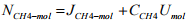= -163.746 + 9.924*6.5 mol/m2s = -99.24 mol/m2s

The document Introduction To Mass Transfer Operation Notes | Study Mass Transfer - Chemical Engineering is a part of the Chemical Engineering Course Mass Transfer.
All you need of Chemical Engineering at this link: Chemical Engineering

## Mass Transfer

29 videos|45 docs|44 tests
 Use Code STAYHOME200 and get INR 200 additional OFF

## Mass Transfer

29 videos|45 docs|44 tests

Track your progress, build streaks, highlight & save important lessons and more!

,

,

,

,

,

,

,

,

,

,

,

,

,

,

,

,

,

,

,

,

,

;